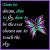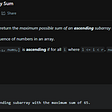# Merge Sort Algorithm in Python

1. Merge sort is efficient to use when the data set is very large. If the array size is smaller, you can use quicksort.
2. Merge sort is a stable algorithm. It means that this sort preserves the sorting of the other dependent columns. Please refer to https://www.coursera.org/lecture/algorithms-part1/stability-pvvLZ for more information.
3. The time complexity is O(n logn) and the space complexity is O(n).
4. More suitable for the linked list than arrays.
`def merge_sort(arr):    arr_len = len(arr)    if(arr_len > 1):        mid = arr_len//2        left_arr = arr[:mid]        right_arr = arr[mid:]        merge_sort(left_arr)        merge_sort(right_arr)        i = j = k = 0        left_arr_len = len(left_arr)        right_arr_len = len(right_arr)        while(i<left_arr_len and j<right_arr_len):            if(left_arr[i] < right_arr[j]):                arr[k] = left_arr[i]                i += 1            else:                arr[k] = right_arr[j]                j += 1            k += 1        if(i < left_arr_len):            arr[k:] = left_arr[i:]        else:            arr[k:] = right_arr[j:]         return arr    return arrarr = [2,4,5,6,2,4,5,6]print(merge_sort(arr))"test cases""empty list []""list with one element ""already sorted list [1,2,3,4,5]""reverse sorted list [7,6,5,4]""list with duplicate values [1,2,2,0,8,7]"`

--

--

--

## More from Abinaya Rajesh

Love podcasts or audiobooks? Learn on the go with our new app.

## Making life easier with immutable deployment## How to range rows in SQL.## Micronaut security attributes — an extra way to secure your endpoints## Authentication with Google Using Xamarin Forms. 403 That’s an error, disallowed_useragent## Dogfooding at RudderStack: Our Data Stack## Building a Marauder’s Map## Overcoming Defeatist Attitudes in Your Life and Developing a Possibility Mindset## LeetCode 1800. Maximum Ascending Subarray — Python Solution## Binary Tree Traversal Without Recursion## Redirecting standard input output to a file in python## LeetCode #167 | Two Sum II — Input Array Is Sorted (Python)